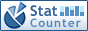Home PC Games Linux Windows Database Network Programming Server Mobile
 Home \ Programming \ Sorting algorithm of dichotomy (binary) insertion sort algorithm - Windows7 system using Vagrant to build Linux virtualized development environment (Linux) - Linux RPM (Linux) - Linux - Common process the command (Linux) - OpenSSL Introduction and compilation steps on Windows, Linux, Mac systems (Linux) - About Java 7 module system (Programming) - Running into the site-wide HTTPS (Server) - ORA-08102 errors (Database) - CentOS7 installed VMware 10 (Linux) - Network security system (Network) - Debian SSD ext4 4K aligned (Linux) - Kali Linux virtualbox rc = Error 1908 workaround (Linux) - Android Touch message passing mechanism analysis (Programming) - To install and deploy Apache under the CentOS (Server) - How to use the command line to obtain Freely RSS source on Linux (Linux) - C ++ string in the end (Programming) - C ++ Supplements - malloc free and new delete the same and different (Programming) - Oracle table space is too large processing time (Database) - You know the difference between URL, URI and URN among you (Linux) - 64-bit Windows Server 2012 R2 install Oracle 10g Second Edition (Database) - NAT and firewall under Linux (Linux) Sorting algorithm of dichotomy (binary) insertion sort algorithm Add Date : 2017-01-08 The basic idea The basic idea of binary insertion sort and direct insertion sort, as in the insertion section i (i>=1) elements, the elements of the previous i-1 has been sorted. The difference is that different methods to find the insertion position, binary insertion sort is to use binary search to find the insertion position. Binary search of the basic idea is: to be interpolated value of the element to find the current value of the intermediate element of the sequence is compared to the current search intermediate element of a sequence as a dividing line, is determined to be inserted element is to find sequence in the current left or right, If it is in its left, places the left to find a sequence for the current sequence, similar to the right. According to the above method, recursively processing the new sequence, until the current search length sequence is less than 1 lookup process ends. Code In the array a, and the left and right borders // row data to be stored in the column to be sorted public void BinaryInsertSort (int [] a, int left, int right) {     int low, middle, high;         int temp;     for (int i = left + 1; i < = right; i ++) {             temp = a [i];             low = left;             high = i - 1;             while (low < = high) {                 middle = (low + high) / 2;                 if (a [i] < a [middle])                     high = middle - 1;                 else                     low = middle + 1;         }             for (int j = i - 1; j> = low; j--)                     a [j + 1] = a [j];             a [low] = temp;         } } Performance Analysis time complexity Binary insertion sort suitable for recording a few more scenes, compared with the direct insertion sort, insertion sort binary spent looking for the insertion position above time is greatly reduced, but the binary insertion sort in terms of the number of mobile recording and direct insertion sort is the same, so its time complexity is O (n2). Second, the record number of comparisons binary insertion sort has nothing to do with the initial sequence. Because each trip to find the sort binary insertion position, a binary number is certain, a binary comparison is necessary only once, so the number of comparisons is certain. Space complexity Like with direct insertion sort, is O (1). Stability Binary insertion sort is a stable sort algorithm. More:
 CopyRight 2002-2022 newfreesoft.com, All Rights Reserved.